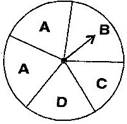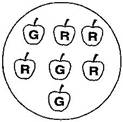# Class 8 NCERT Solutions – Chapter 5 Data Handling – Exercise 5.3

• Last Updated : 05 Nov, 2020

### Question 1. List the outcomes you can see in these experiments.

(a) Spinning a wheel (b) Tossing two coins togetherSolution:

(a) Since, there are four letters A, B, C and D in a spinning wheel therefore there are 4 possible outcomes.

(b) When two coins are tossed together, there are four possible outcomes HH, TT, HT and TH.

### Question 2. When a die is thrown, list the outcomes of an event of getting

(i) (a) a prime number (b) not a prime number.

(ii) (a) a number greater than 5 (b) a number not greater than 5.

Solution:

(i) (a) Outcomes of event of getting a event with prime number are 2, 3 and 5.

(b) Outcomes of event of not getting a event with prime number are 1, 4 and 6.

(ii) (a) Outcomes of event of getting a event with number greater than 5 is 6.

(b) Outcomes of event of not getting a event with number greater than 5 are 1, 2, 3, 4 and 5.

### Question 3. Find the

(a) Probability of the pointer stopping on D in (Question 1-(a))?

(b) Probability of getting an ace from a well shuffled deck of 52 playing cards?

(c) Probability of getting a red apple. (See figure below)Solution:

(a) In a spinning wheel, there are five pointers A, A, B, C and D.

Since there are five outcomes from which the Pointer stops at D. So D is the outcome.

Therefore, the probability of the pointer stop on D =1/5

(b) There are 4 aces in a deck of 52 playing cards(one from each suit i.e. diamond, club , heart and spade).

Since, there are four events of getting an ace. Therefore, probability of getting an ace = 4/52 = 1/13

(c) Total number of apples are 7, out of which number of red and green are 4 and 3 respectively.

Probability of getting a red apple = 4/7

### Question 4. Numbers 1 to 10 are written on ten separate slips (one number on one slip), kept in a box and mixed well. One slip is chosen from the box without looking into it. What is the probability of

(i) getting a number 6?

(ii) getting a number less than 6?

(iii) getting a number greater than 6?

(iv) getting a 1-digit number?

Solution:

Given, there are 10 separate slips in the box.

(i) Number 6 is written only on 1 slip.

Therefore, probability of getting a number 6 is = 1/10

(ii) Numbers less than 6 are 1, 2, 3, 4 and 5 which are 5. Since there are 5 outcomes.

Therefore, probability of getting a number less 6 is = 5/10 = 1/2

(iii) Number greater than 6 out of 10 are 7, 8, 9, 10. Since there are 4 possible outcomes.

Therefore, probability of getting a number greater than 6 is = 4/10 = 2/5

(iv) Since one-digit numbers are 1, 2, 3, 4, 5, 6, 7, 8, 9 out of 10.

Therefore, probability of getting a 1-digit number is = 9/10

### Question 5. If you have a spinning wheel with 3 green sectors, 1 blue sector and 1 red sector, what is the probability of getting a green sector? What is the probability of getting a non-blue sector?

Solution:

A total of 5 sectors are present(3 green + 1 blue + 1 red).

Out of the 5 sectors, 3 sectors are green Therefore, the probability of getting a green sector = 3/5

Out of the 5 sectors, 1 sector is blue. Therefore, Non-blue sectors=5 – 1 = 4 sectors

Therefore, probability of getting a non-blue sector is = 4/5

### Question 6. Find the probabilities of the events given in Question 2.

Solution:

When a die is thrown, there are total six possible outcomes, i.e., 1, 2, 3, 4, 5 and 6.

(i) (a) 2, 3 and 5 are the prime numbers. So there are 3 outcomes out of 6.

Therefore, probability of getting a prime number is = 3/6 =1/2

(b) 1, 4 and 6 are not the prime numbers. So there are 3 outcomes out of 6.

Therefore probability of getting a prime number is = 3/6 = 1/2

(ii) (c) Only 6 is greater than 5.

Since there is 1 outcome out of 6 possible outcomes.

Therefore, probability of getting a number greater than 5 is = 1/6

(d) Number which are not greater than 5 are 1, 2, 3, 4 and 5. Since there are 5 outcomes out of 6.

Therefore, probability of not getting a number greater than 5 is = 5/6

My Personal Notes arrow_drop_up